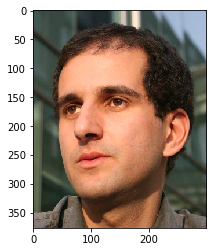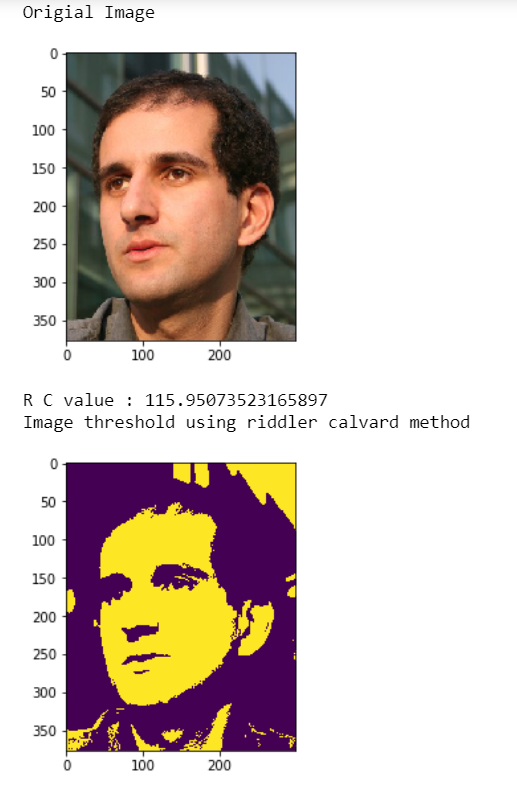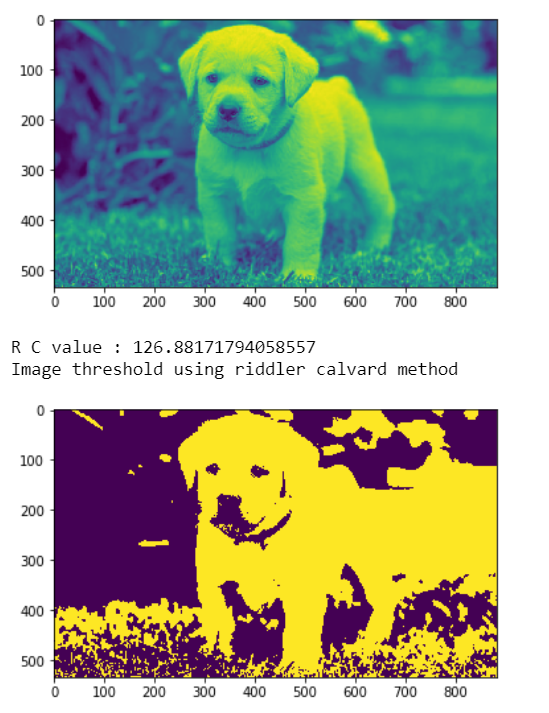Skip to content
Related Articles
Mahotas – Riddler-Calvard Method
• Last Updated : 10 Jun, 2021

In this article we will see how we can implement riddler calvard method in mahotas. This is alternative of otsu’s method. The Ridler and Calvard algorithm uses an iterative clustering approach. First a initial estimate of the threshold is to be made (e.g. mean image intensity). Pixels above and below the threshold are assigned to the object and background classes respectively.
In this tutorial we will use “luispedro” image, below is the command to load it.

`mahotas.demos.load('luispedro')`

Below is the luispedro imageIn order to do this we will use mahotas.rc method

Syntax : mahotas.rc(image)
Argument : It takes image object as argument
Return : It returns numpy.float64

Note : Input image should be filtered or should be loaded as grey
In order to filter the image we will take the image object which is numpy.ndarray and filter it with the help of indexing, below is the command to do this

`image = image[:, :, 0]`

Example 1:

## Python3

 `# importing required libraries``import` `mahotas``import` `mahotas.demos``import` `numpy as np``from` `pylab ``import` `imshow, gray, show``from` `os ``import` `path` `# loading the image``photo ``=` `mahotas.demos.load(``'luispedro'``)` `# showing original image``print``(``"Origial Image"``)``imshow(photo)``show()` `# loading image as grey``photo ``=` `mahotas.demos.load(``'luispedro'``, as_grey ``=` `True``)` `# converting image type to unit8``# beacuse as_grey returns floating values``photo ``=` `photo.astype(np.uint8)` `# riddler calvard``T_rc ``=` `mahotas.rc(photo)` `# printing otsu value``print``(``"R C value : "` `+` `str``(T_rc))` `print``(``"Image threshold using riddler calvard method"``)``# showing image``# image values should be greater than T_rc value``imshow(photo > T_rc)``show()`

Output :Example 2:

## Python3

 `# importing required libraries``import` `mahotas``import` `numpy as np``from` `pylab ``import` `imshow, show``import` `os`  `# loading iamge``img ``=` `mahotas.imread(``'dog_image.png'``)``    `  `# setting filter to the image``img ``=` `img[:, :, ``0``]` `imshow(img)``show()`  `# riddler calvard``T_rc ``=` `mahotas.rc(img)` `# printing otsu value``print``(``"R C value : "` `+` `str``(T_rc))` `print``(``"Image threshold using riddler calvard method"``)``# showing image``# image values should be greater than T_rc value``imshow(img > T_rc)``show()`

Output :Attention geek! Strengthen your foundations with the Python Programming Foundation Course and learn the basics.

To begin with, your interview preparations Enhance your Data Structures concepts with the Python DS Course. And to begin with your Machine Learning Journey, join the Machine Learning – Basic Level Course

My Personal Notes arrow_drop_up